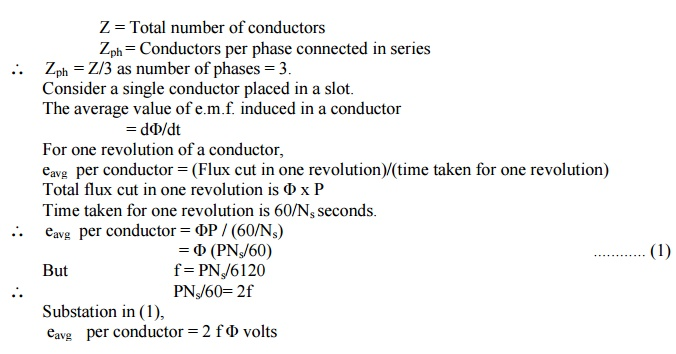Home | | Electrical Engineering and Instrumentation | Equation of Induced EMF - Synchronous Generator or Alternator

# Equation of Induced EMF - Synchronous Generator or Alternator

Electrical Engineering and Instrumentation - Induction Machines and Synchronous Machines

Equation of Induced EMF

Let          Φ = Flux per pole, in Wb

P = Number of poles

Ns = Synchronous speed in r.p.m.

f = Frequency of induced e.m.f. in HzAssume full pitch winding for simplicity i.e. this conductor is connected to a conductor which is 180o electrical apart. So there two e.m.f.s will try to set up a current in the same direction i.e. the two e.m.f. are helping each other and hence resultant e.m.f. per turn will be twice the e.m.f. induced in a conductor.

... E.m.f. per turn = 2 x (e.m.f. per conductor)

= 2 x (2 f Φ)

= 4 f Φ volts

Let Tph be the total number of turn per phase connected in series. Assuming concentrated winding, we can say that all are placed in single slot per pole per phase. So induced e.m.f.s in all turns will be in phase as placed in single slot. Hence net e.m.f. per phase will be algebraic sum of the e.m.f.s per turn.

Average Eph = Tph x (Average e.m.f. per turn)

Average Eph = Tph x 4 f Φ

But in a.c. circuits R.M.S. value of an alternating quantity is used for the analysis. The form factor is 1.11 of sinusoidal e.m.f.

Kf = (R.M.S.)/Average = 1.11     ......... for sinusoidal

...  R.M.S. value of Eph   = K x Average value

E = 4.44 x f Φ Tph  volts

Study Material, Lecturing Notes, Assignment, Reference, Wiki description explanation, brief detail
Electrical Engineering and Instrumentation : Induction Machines and Synchronous Machines : Equation of Induced EMF - Synchronous Generator or Alternator |